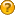###Author Topic: Importing Excel Data to Drive a StateMachine Diagram using an Activity Diagram  (Read 117 times)

#### CMcD

• EA Novice
•• Posts: 6
• Karma: +0/-0##### Importing Excel Data to Drive a StateMachine Diagram using an Activity Diagram
« on: July 31, 2020, 09:52:50 pm »
Hi all,

I'm trying to learn the basics of simulating Activity / StateMachine diagrams along with signalling and transitions

I'm trying to create a basic tool that can do a few main functions
1. It can access an Excel file to assign a value from the Excel spreadsheet to an Attribute value in EA

<img src="https://i.ibb.co/WW04yhh/Excel-Data.png" alt="Excel-Data" border="0">

2. It can make a decision based off the value of an Attribute to decide which Actions are taken in an Activity diagram (i.e. using Guards)

<img src="https://i.ibb.co/smZqqpL/Activity.png" alt="Activity" border="0">

3. The signals sent by the activity diagram cause a StateMachine diagram to switch states

<img src="https://i.ibb.co/kxHL4DX/State.png" alt="State" border="0">

I am having trouble with the first and third functions

For the first:
 I know you can import a CSV to EA, but is there any way that EA can carry out an action during a simulation that directly accesses a CSV file without having to manually import it? - i.e. can the simulation be run in real time
 If that can't be done, how would the CSV file be structured in such a way that when imported, it imports and creates Attributes with the relevant values to allow the aforementioned Activity diagram decision making

For the third:
 How is it that I get a signal sent in the Activity diagram to be transferred and received by the StateMachine diagram such that the two diagrams both simulate together and represent the dynamic behaviour of the system
 Would this involve the use of ports between Blocks that contain either diagram, or is there another suitable approach
 Or would I have to make use of the "target" Action Pins, but I am unsure as to how to actually use them

<img src="https://i.ibb.co/8xpV91c/Blocks.png" alt="Blocks" border="0">

Thanks